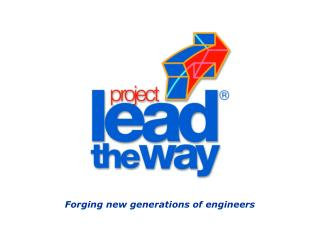DownloadDownload PresentationForging new generations of engineers

# Forging new generations of engineers

Download Presentation## Forging new generations of engineers

- - - - - - - - - - - - - - - - - - - - - - - - - - - E N D - - - - - - - - - - - - - - - - - - - - - - - - - - -
##### Presentation Transcript

1. Forging new generations of engineers

2. Lever and Linkage Systems Methods of Power Transmission

3. The 3 Classes of Levers • Each class has the fulcrum, effort and load arranged in a different way. • The three classes are:

4. Class 1 Lever • Fulcrum between the load and the effort

5. Class 2 Lever • Load between the fulcrum and the effort

6. Class 3 Lever • Effort is between load and fulcrum

7. ROCKER ARM • Has an input and output arm which rock around a fulcrum point • Acts as a link which transmit force or motion between moving parts • Operate usually as a first class lever • Change linear input motion to an opposed linear motion

8. BELL CRANK • A bent first class lever that pivots at the bent point or elbow • Used to change the direction of force or motion 90 degrees or less • Used to convert rotary motion to straight line or reciprocating motion

9. BELL CRANK • Reciprocating motion Top • pin A moves slider to right • pin B moves bell crank which moves slider to left Bottom • pins move crank; spring returns to start position

10. DOUBLE BELL CRANK • PUSH, PULL TYPE PUSH • requires stiff connecting rods PULL • can use flexible wires,cables, ropes

11. DIFFERENTIAL LINKAGE • Used to combine several motion inputs into a resulting output • Three pivot points, none of which are fixed but are free to float within limits • Any pivot can function as an input • A motion control mechanism rather than a means of transmitting force or power

13. FOUR-BAR LINKAGES • To make a four-bar linkage a usable device, one of the four links, or one of the pivots, must be fixed • Depending on the fixed pivot or link different motion outputs will occur

15. FOUR-BAR LINKAGES • FIXED PIVOT • the point opposite the the fixed point is normally the output point • the remaining two pivot points are usually the input points

16. PARALLEL LINKAGE • To provide parallel motion of a line or a surface as the linkage moves about two controlled pivot points • The arms remain parallel through the operation of the connecting link Eg: man lift, robot gripper

17. SCISSOR LINKAGE • To change the direction of a linear motion input to a linear motion output that is perpendicular to the input • Large motion output (distance advantage) with a small motion input • Especially true of a series of connecting linkages • One fixed pivot point

18. SCISSOR LINKAGE • Two pair of links of different length • Less force at input pivot point - close to pivot point • output is identical

19. SCISSOR LINKAGE • Two pair of links of different length • More force at input pivot point - farther from pivot point • output is identical

20. WATT STRAIGHT LINE MOTION • Does not form a parallelogram but consists of two equal length pivoting links and a connecting link • Produces a straight line motion in the connecting link